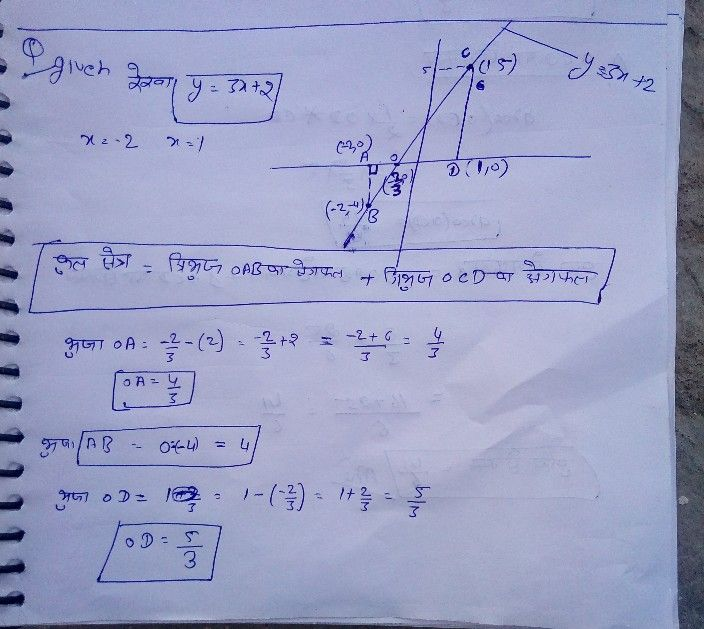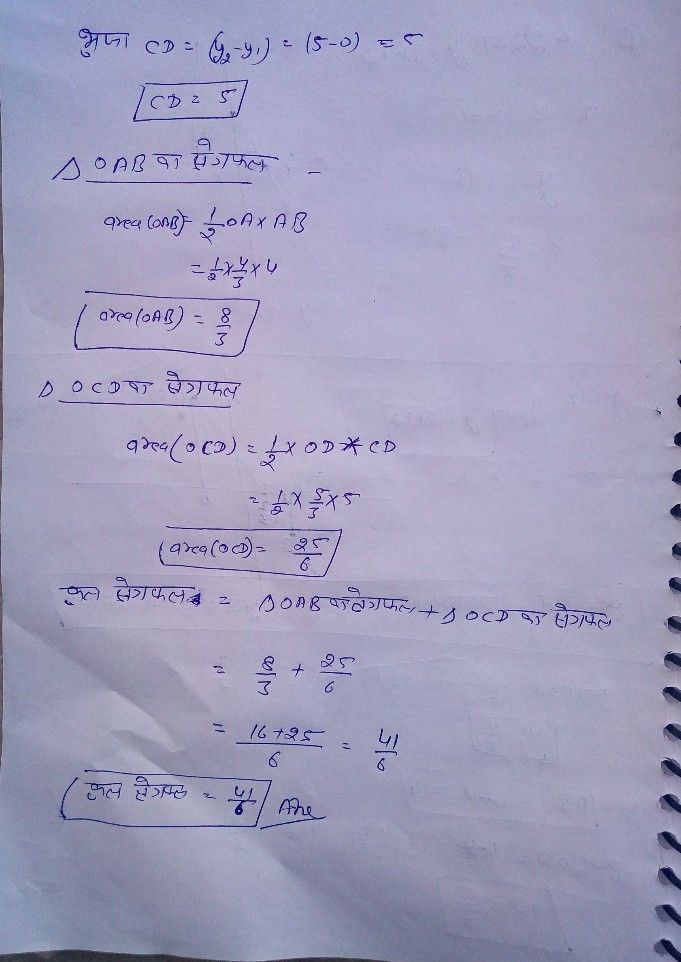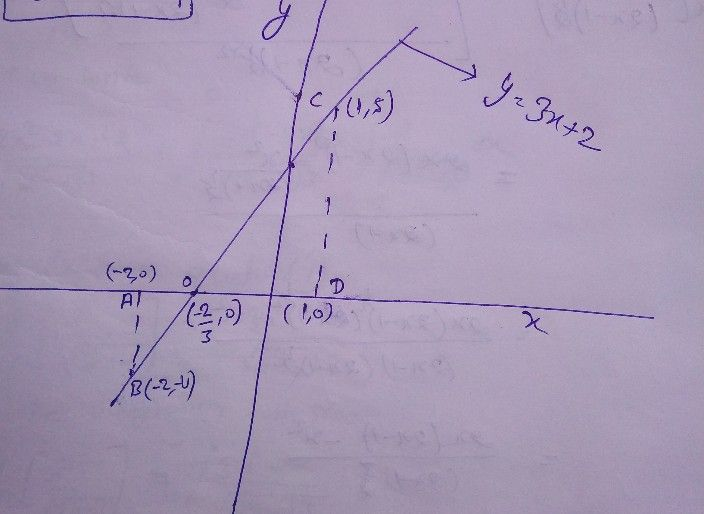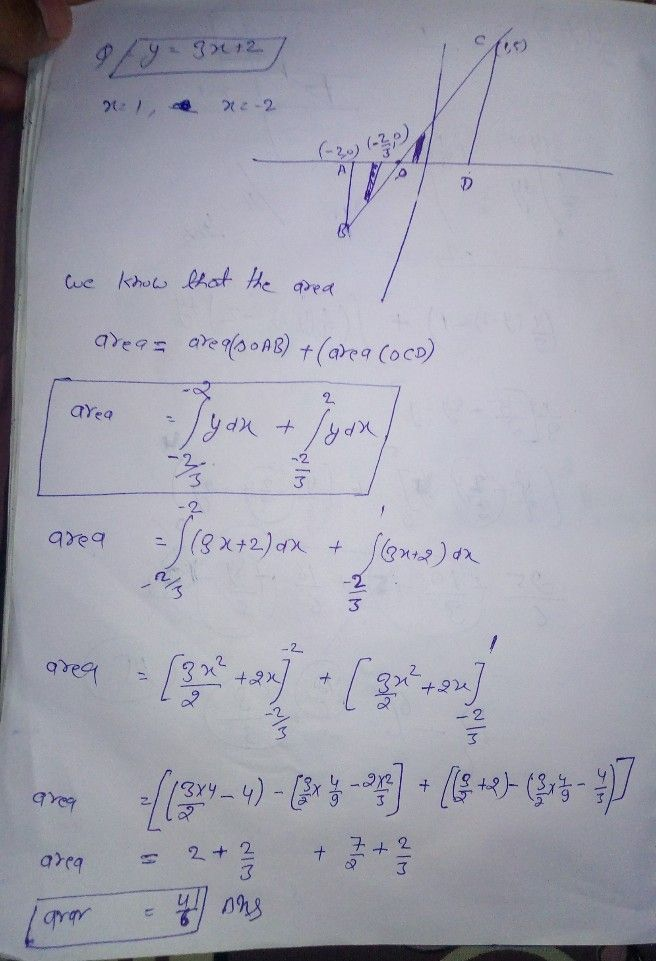Symbol
Problem$3.$ al $y=3x+2,x-$ $x-378,$ $x=-2$ 2 a $x=1$ a a aa l Find the area enclosed by the line $y=3x+2,x=-2,x=1$ & $X$ axis. $→$ $△$ $△$ $△$ $→$ $△$ A A TOT $→$
Calculus
SolutionStudent
hello sir i need it in english and it is a question of calculus so we must use integration